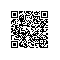# 文本比较算法Ⅲ——计算文本的相似度

在“文本比较算法Ⅱ——Needleman/Wunsch算法”中，介绍了最长公共子串的计算。

在给定的字符串A和字符串B，LD(A,B)表示编辑距离，LCS(A,B)表示最长公共子串的长度。

如何来度量它们之间的相似度呢？

不妨设S(A,B)来表示字符串A和字符串B的相似度。那么，比较合理的相似度应该满足下列性质。

性质一：0≤S(A,B)≤100%，0表示完全不相似，100%表示完全相等

性质二：S(A,B)=S(B,A)

目前，网上介绍的各种相似度的计算，都有各自的不尽合理的地方。

计算公式一：S(A,B)=1/(LD(A,B)+1)

能完美的满足性质二。

当LD(A,B)=0时，S(A,B)=100%，不过无论LD(A,B)取任何值，S(A,B)＞0，不能满足性质一。

计算公式二：S(A,B)=1－LD(A,B)/Len(A)

当Len(B)＞Len(A)时，S(A,B)＜0。不满足性质一。

有人会说，当S(A,B)<0时，强制指定S(A,B)=0就解决问题了。

问题是，S(A,B)=1－LD(A,B)/Len(A)，而S(B,A)=1－LD(B,A)/Len(B)。当Len(A)≠Len(B)时，S(A,B)≠S(B,A)。不满足性质二

还有一个例子可以说明问题

A="BC"，B="CD"，C="EF"

S(A,B)=1－LD(A,B)/Len(A)=1－2/2=0

S(A,C)=1－LD(A,C)/Len(A)=1－2/2=0

A和B的相似度与A和C的相似度是一样的。不过很明显的是B比C更接近A

计算公式三：S(A,B)=LCS(A,B)/Len(A)

这个公式能完美的满足的性质一

不过当Len(A)≠Len(B)时，S(A,B)≠S(B,A)。不满足性质二

用一个例子说明问题

A="BC"，B="BCD"，C="BCEF"

S(A,B)=LCS(A,B)/Len(A)=2/2=100%

S(A,C)=LCS(A,C)/Len(A)=2/2=100%

A和B的相似度与A和C的相似度是一样的。不过很明显的是B比C更接近A

上面是网上能找到的三个计算公式，从上面的分析来看都有各自的局限性。

我们看一个例子：

A=GGATCGA，B=GAATTCAGTTA，LD(A,B)=5，LCS(A,B)=6

他们的匹配为：

A：GGA_TC_G__A

B：GAATTCAGTTA

可以看出上面蓝色的部分表示的是LCS部分，黑色表示的是LD部分。

因此，给出一个新的公式

S(A,B)=LCS(A,B)/(LD(A,B)+LCS(A,B))

这个公式能解决上述三个公式的种种不足。

而LD(A,B)+LCS(A,B)表示两个字符串A、B的最佳匹配字串的长度。这个是唯一的。

还有注意的是LD(A,B)+LCS(A,B)和Max(Len(A),Len(B))这两个并不完全相等。

本文转自万仓一黍博客园博客，原文链接：http://www.cnblogs.com/grenet/archive/2010/06/04/1751147.html，如需转载请自行联系原作者使用钉钉扫一扫加入圈子
+ 订阅